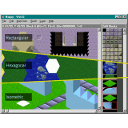# sourceafFantomMappy::Layer.fan

```using gfx::Size

** Represents one of the layers in a `MappyMap`.
** See `LayerViewer` for details on rendering a 'Layer' to the screen.
@Js
class Layer {
private Block[]         blocks
private AnimBlock[]     animBlocks
private LayerData       layerData

** The size of the layer in blocks.
const   Size            sizeInBlocks

** The size of the layer in pixels.
const   Size            sizeInPixels

** Creates a 'Layer'.
this.layerData      = layerData
this.blocks         = blocks
this.animBlocks     = animBlocks
this.sizeInBlocks   = layerData.size
this.sizeInPixels   = mapHeader.mapSizeInPixels // TODO: is this correct?
}

** Returns the `Block` at the given block coordinates.
**
** If the block is an animation block then the current block in the anim sequence is returned.
Block blockAt(Int blockX, Int blockY) {
blockIndex := isAnimBlock(blockX, blockY) ? animBlockAt(blockX, blockY).frame : layerData.get(blockX, blockY)
return blocks[blockIndex]
}

** Returns the `AnimBlock` at the given block coordinates.
**
** If the block is not an `AnimBlock` an 'ArgErr' is thrown.
AnimBlock animBlockAt(Int blockX, Int blockY) {

// grab the index...
blockIndex := layerData.get(blockX, blockY)

// is it an animation block?
if (blockIndex >= 0)
throw ArgErr("The block at coors [\$blockX][\$blockY] is not an AnimBlock")

// ...and return the anim block
return animBlocks[-blockIndex - 1]
}

** Checks if the block at the given coordinates is an `AnimBlock`.
Bool isAnimBlock(Int blockX, Int blockY) {
return layerData.get(blockX, blockY) < 0
}

** Performs a collision detection test at the given pixel coordinates using the `Block`s
** collision flags.
Bool isCollisionAt(Int pixelX, Int pixelY) {
collisionAt(pixelX, pixelY) != null
}

** Performs a collision detection test at the given pixel coordinates using the `Block`s
** collision flags. Returns null if n collision occurred.
BlockCorner? collisionAt(Int pixelX, Int pixelY) {

// note this method isn't in the Block because a block has no notion
// of it's size - i guess it should do but I'm thinking of code size
blockX      := pixelX / blockSize.w
blockY      := pixelY / blockSize.h
modX        := pixelX % blockSize.w
modY        := pixelY % blockSize.h
block       := blockAt(blockX, blockY)

BlockCorner? corner

if (modX >= (blockSize.w / 2))
if (modY >= (blockSize.h / 2))
corner = BlockCorner.bottomRight
else
corner = BlockCorner.topRight
else
if (modY >= (blockSize.h / 2))
corner = BlockCorner.bottomLeft
else
corner = BlockCorner.topLeft

if (!block.collisionFlag[corner])
corner = null

return corner
}
}

** Represents the data in a `Layer`. It's essentially a 2D array of 'Ints'.
@Js
class LayerData {
internal const  Size    size
private Int[]   data    := [,]

internal new make(Size layerSize) {
this.size = layerSize
data.fill(0, size.w * size.h)
}

** Gets the data at the given coordinates.
** Throws 'ArgErr' should the coordinates be out of bounds.
Int get(Int x, Int y) {
checkXY(x, y)
index := (y * size.w) + x
return data[index]
}

** Sets the data at the given coordinates.
** Throws 'ArgErr' should the coordinates be out of bounds.
Void set(Int x, Int y, Int val) {
checkXY(x, y)
index := (y * size.w) + x
data[index] = val
}

internal Void div(Int x, Int y, Int divVal) {
checkXY(x, y)
index := (y * size.w) + x
data[index] /= divVal
}

private Void checkXY(Int x, Int y) {
if (x < 0)          throw ArgErr("X '\$x' can not be < 0")
if (x >= size.w)    throw ArgErr("X '\$x' can not be >= \$size.w")
if (y < 0)          throw ArgErr("Y '\$y' can not be < 0")
if (y >= size.h)    throw ArgErr("Y '\$y' can not be >= \$size.h")
}
}
```